# Title 40

## SECTION 53.33

### 53.33 Test Procedure for Methods for Lead (Pb).

§ 53.33 Test Procedure for Methods for Lead (Pb).

(a) General. The reference method for Pb in TSP includes two parts, the reference method for high-volume sampling of TSP as specified in 40 CFR 50, appendix B and the analysis method for Pb in TSP as specified in 40 CFR 50, appendix G. Correspondingly, the reference method for Pb in PM 10 includes the reference method for low-volume sampling of PM 10 in 40 CFR 50, appendix O and the analysis method of Pb in PM 10 as specified in 40 CFR 50, appendix Q. This section explains the procedures for demonstrating the equivalence of either a candidate method for Pb in TSP to the high-volume reference methods, or a candidate method for Pb in PM 10 to the low-volume reference methods.

(1) Pb in TSP - A candidate method for Pb in TSP specifies reporting of Pb concentrations in terms of standard temperature and pressure. Comparisons of candidate methods to the reference method in 40 CFR 50, appendix G must be made in a consistent manner with regard to temperature and pressure.

(2) Pb in PM 10 - A candidate method for Pb in PM 10 must specify reporting of Pb concentrations in terms of local conditions of temperature and pressure, which will be compared to similarly reported concentrations from the reference method in 40 CFR 50 appendix Q.

(b) Comparability. Comparability is shown for Pb methods when the differences between:

(1) Measurements made by a candidate method, and

(2) Measurements made by the reference method on simultaneously collected Pb samples (or the same sample, if applicable), are less than or equal to the values specified in table C-3 of this subpart.

(c) Test measurements. Test measurements may be made at any number of test sites. Augmentation of pollutant concentrations is not permitted, hence an appropriate test site or sites must be selected to provide Pb concentrations in the specified range.

(d) Collocated samplers. The ambient air intake points of all the candidate and reference method collocated samplers shall be positioned at the same height above the ground level, and between 2 meters (1 meter for samplers with flow rates less than 200 liters per minute (L/min)) and 4 meters apart. The samplers shall be oriented in a manner that will minimize spatial and wind directional effects on sample collection.

(e) Sample collection. Collect simultaneous 24-hour samples of Pb at the test site or sites with both the reference and candidate methods until at least 10 sample pairs have been obtained.

(1) A candidate method for Pb in TSP which employs a sampler and sample collection procedure that are identical to the sampler and sample collection procedure specified in the reference method in 40 CFR part 50, Appendix B, but uses a different analytical procedure than specified in 40 CFR Appendix G, may be tested by analyzing pairs of filter strips taken from a single TSP reference sampler operated according to the procedures specified by that reference method.

(2) A candidate method for Pb in PM 10 which employs a sampler and sample collection procedure that are identical to the sampler and sample collection procedure specified in the reference method in 40 CFR part 50, Appendix O, but uses a different analytical procedure than specified in 40 CFR Appendix Q, requires the use of two PM 10 reference samplers because a single 46.2-mm filter from a reference sampler may not be divided prior to analysis. It is possible to analyze a 46.2-mm filter first with the non-destructive X-ray Fluorescence (XRF) FRM and subsequently extract the filter for other analytical techniques. If the filter is subject to XRF with subsequent extraction for other analyses, then a single PM 10 reference sampler may be used for sample collection.

(3) A candidate method for Pb in TSP or Pb in PM 10 which employs a direct reading (e.g., continuous or semi-continuous sampling) method that uses the same sampling inlet and flow rate as the FRM and the same or different analytical procedure may be tested. The direct measurements are then aggregated to 24-hour equivalent concentrations for comparison with the FRM. For determining precision in section (k), two collocated direct reading devices must be used.

(f) Audit samples. Three audit samples must be obtained from the address given in § 53.4(a). For Pb in TSP collected by the high-volume sampling method, the audit samples are 3/4 × 8-inch glass fiber strips containing known amounts of Pb in micrograms per strip (µg/strip) equivalent to the following nominal percentages of the National Ambient Air Quality Standard (NAAQS): 30%, 100%, and 250%. For Pb in PM 10 collected by the low-volume sampling method, the audit samples are 46.2-mm polytetrafluorethylene (PTFE) filters containing known amounts of Pb in micrograms per filter (µg/filter) equivalent to the same percentages of the NAAQS: 30%, 100%, and 250%. The true amount of Pb (Tqi), in total µg/strip (for TSP) or total µg/filter (for PM 10), will be provided for each audit sample.

(g) Filter analysis. (1) For both the reference method samples (e) and the audit samples (f), analyze each filter or filter extract three times in accordance with the reference method analytical procedure. This applies to both the Pb in TSP and Pb in PM 10 methods. The analysis of replicates should not be performed sequentially, i.e., a single sample should not be analyzed three times in sequence. Calculate the indicated Pb concentrations for the reference method samples in micrograms per cubic meter (µg/m 3) for each analysis of each filter. Calculate the indicated total Pb amount for the audit samples in µg/strip for each analysis of each strip or µg/filter for each analysis of each audit filter. Label these test results as R1A, R1B, R1C, R2A, R2B, etc., Q1A, Q1B, Q1C, etc., where R denotes results from the reference method samples; Q denotes results from the audit samples; 1, 2, 3 indicate the filter number, and A, B, C indicate the first, second, and third analysis of each filter, respectively.

(2) For the candidate method samples, analyze each sample filter or filter extract three times and calculate, in accordance with the candidate method, the indicated Pb concentration in µg/m 3 for each analysis of each filter. The analysis of replicates should not be performed sequentially. Label these test results as C1A, C1B, C2C, etc., where C denotes results from the candidate method. For candidate methods which provide a direct reading or measurement of Pb concentrations without a separable procedure, C1A = C1B = C1C, C2A = C2B = C2C, etc.

(h) Average Pb concentration. For the reference method, calculate the average Pb concentration for each filter by averaging the concentrations calculated from the three analyses as described in (g)(1) using equation 1 of this section: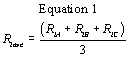Where, i is the filter number.

(i) Analytical Bias. (1) For the audit samples, calculate the average Pb concentration for each strip or filter analyzed by the reference method by averaging the concentrations calculated from the three analyses as described in (g)(1) using equation 2 of this section: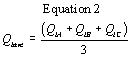Where, i is audit sample number.

(2) Calculate the percent difference (Dq) between the average Pb concentration for each audit sample and the true Pb concentration (Tq) using equation 3 of this section: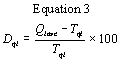(3) If any difference value (Dqi) exceeds ±5 percent, the bias of the reference method analytical procedure is out-of-control. Corrective action must be taken to determine the source of the error(s) (e.g., calibration standard discrepancies, extraction problems, etc.) and the reference method and audit sample determinations must be repeated according to paragraph (g) of this section, or the entire test procedure (starting with paragraph (e) of this section) must be repeated.

(j) Acceptable filter pairs. Disregard all filter pairs for which the Pb concentration, as determined in paragraph (h) of this section by the average of the three reference method determinations, falls outside the range of 30% to 250% of the Pb NAAQS level in µg/m 3 for Pb in both TSP and PM 10. All remaining filter pairs must be subjected to the tests for precision and comparability in paragraphs (k) and (l) of this section. At least five filter pairs must be within the specified concentration range for the tests to be valid.

(k) Test for precision. (1) Calculate the precision (P) of the analysis (in percent) for each filter and for each method, as the maximum minus the minimum divided by the average of the three concentration values, using equation 4 or equation 5 of this section: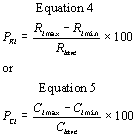Where, i indicates the filter number.

(2) If a direct reading candidate method is tested, the precision is determined from collocated devices using equation 5 above.

(3) If any reference method precision value (PRi) exceeds 15 percent, the precision of the reference method analytical procedure is out-of-control. Corrective action must be taken to determine the source(s) of imprecision, and the reference method determinations must be repeated according to paragraph (g) of this section, or the entire test procedure (starting with paragraph (e) of this section) must be repeated.

(4) If any candidate method precision value (PCi) exceeds 15 percent, the candidate method fails the precision test.

(5) The candidate method passes this test if all precision values (i.e., all PRi's and all PCi's) are less than 15 percent.

(l) Test for comparability. (1) For each filter or analytical sample pair, calculate all nine possible percent differences (D) between the reference and candidate methods, using all nine possible combinations of the three determinations (A, B, and C) for each method using equation 6 of this section: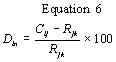Where, i is the filter number, and n numbers from 1 to 9 for the nine possible difference combinations for the three determinations for each method (j = A, B, C, candidate; k = A, B, C, reference).

(2) If none of the percent differences (D) exceeds ±20 percent, the candidate method passes the test for comparability.

(3) If one or more of the percent differences (D) exceed ±20 percent, the candidate method fails the test for comparability.

(4) The candidate method must pass both the precision test (paragraph (k) of this section) and the comparability test (paragraph (l) of this section) to qualify for designation as an equivalent method.

(m) Method Detection Limit (MDL). Calculate the estimated MDL using the guidance provided in 40 CFR, Part 136 Appendix B. It is essential that all sample processing steps of the analytical method be included in the determination of the method detection limit. Take a minimum of seven blank filters from each lot to be used and calculate the detection limit by processing each through the entire candidate analytical method. Make all computations according to the defined method with the final results in µg/m 3. The MDL of the candidate method must be equal to, or less than 5% of the level of the Pb NAAQS.

[73 FR 67057, Nov. 12, 2008]